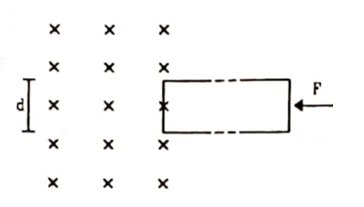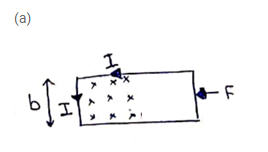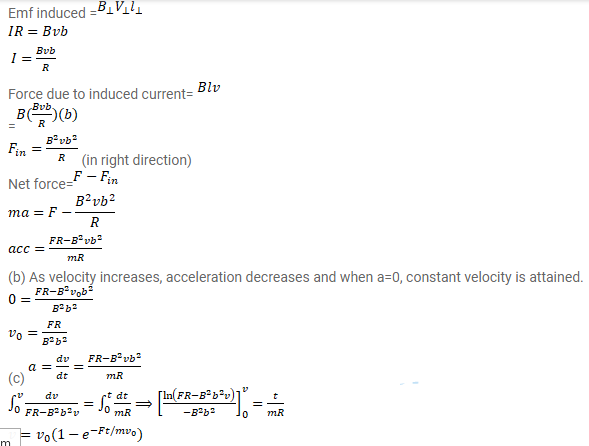# The rectangular wire-frame,

Question:

The rectangular wire-frame, shown in figure has a width $\mathrm{d}$, mass $\mathrm{m}$, resistance $\mathrm{R}$ and a large length. A uniform magnetic field B exists to the left of the frame. A constant force $F$ starts pushing the frame into the magnetic field at $t=0$. (a) Find the acceleration of the frame when its speed has increased to $v$. (b) Show that after some time the frame will move with a constant velocity till the whole frame enters into the magnetic field. Find this velocity ${ }^{v_{0}}$. (c) Show that the velocity at $t$ is given bySolution: